# Calling R from java using JRI

JRI is a Java/R Interface, which allows to run R inside Java. It loads R dynamic library and provide a interface to call R. You can use it to call R function or a running REPL.

By the way, there is another project rJava , which is used to create objects, call methods and access fields of Java objects from R.

JRI uses native library to call R from java. In its website, it provides the compiled library for windows and Mac. If you want the library for Linux, you can compile from the source yourself.

Java Environment setup

So, if you want to use JRI, you needs the JRI.jar and native library (is libjri.jnilib on Mac). The JRI.jar should be in the class path of java, and the native library should be set within java.library.path.

At first, download the JRI package and unzip. Find the JRI.jar and copy the JRI.jar into the project. Of course, you can add the reference from the directory of unzipped package. But, it will be convenient to copy it into the project.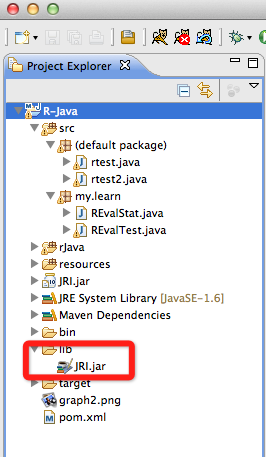And then add this jar to the project build path.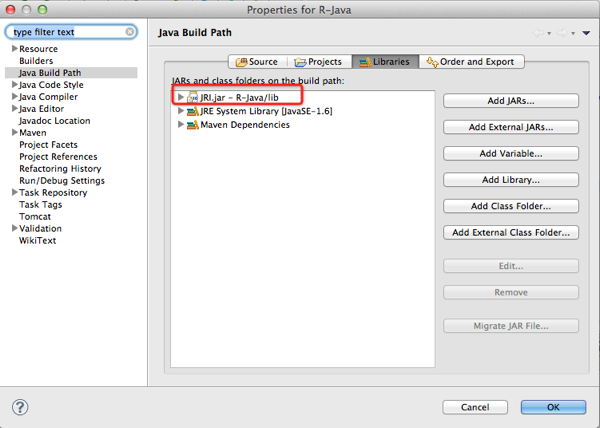In JRI 's package, there are 2 example files rtest.java and rtest2.java. Just copy these 2 files not the project.

To run these java, we need to set the "java.library.path" then the Java can find the native library from there. And of course, we should have R installed and rJava package. The package rJava will provide the native library of JRI. You can install rJava with install.packages('rJava') from R console.

So, in running configuration, in Argument tab, add the system properties of "java.library.path" as below.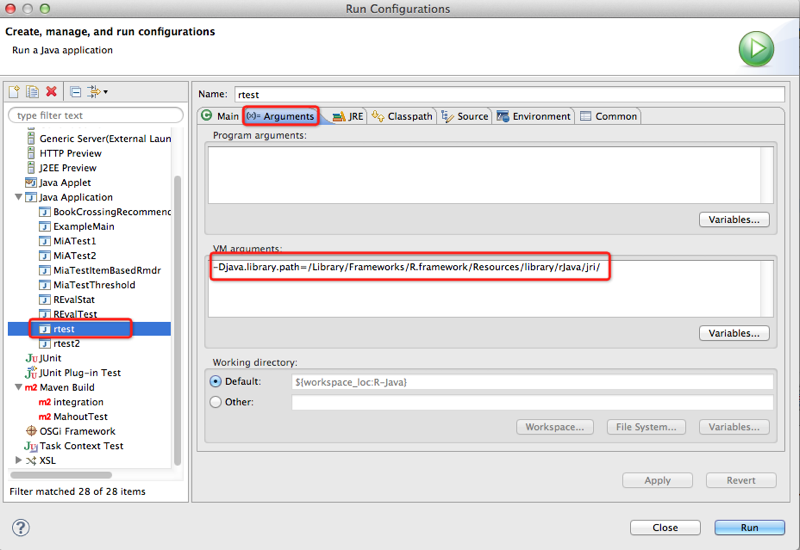The directory:

/Library/Frameworks/R.framework/Resources/library/rJava/jri/

Is the directory of rJava package in R in my mac system. If your system is windows, you can also find it from the R installation directory.

And, click "Run" then you should can run this Java file.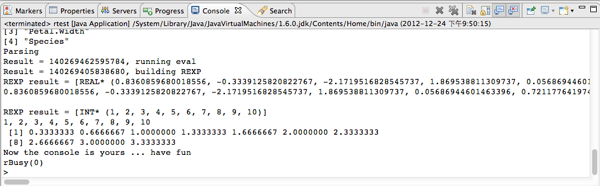If you got any error to say about setting R_Home, it is because that your system can not find the R installation. Then you should set it in eclipse: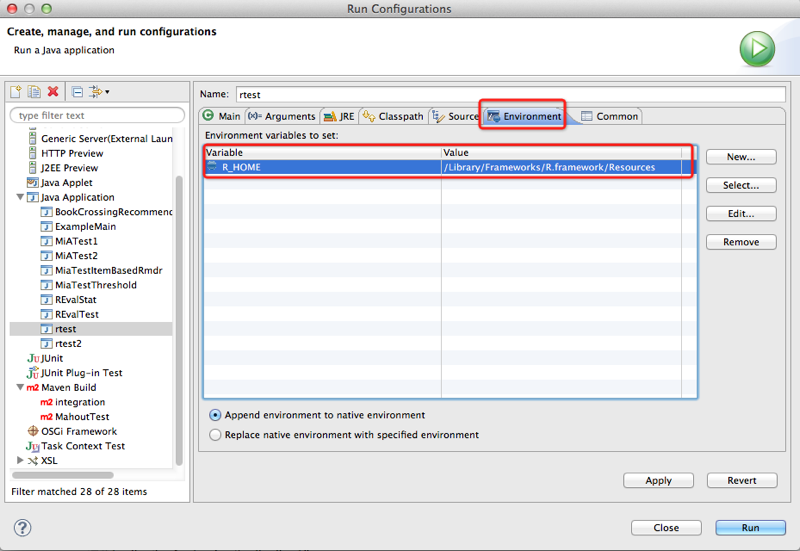In my mac system, it is :/Library/Frameworks/R.framework/Resources . On windows system, it should be like C:\program files\R ...

Call R function in Java

One usage of JRI is to call R functions. The following class demonstrated many kinds of R function calling:

 package my.learn;       import java.util.Enumeration;       import org.rosuda.JRI.REXP;   import org.rosuda.JRI.RList;   import org.rosuda.JRI.RVector;   import org.rosuda.JRI.Rengine;       public class RFunctionTest {           public static void main(String[] args) {         Rengine re = new Rengine(new String[] { "--vanilla" }, false, null);         System.out.println("Rengine created, waiting for R");           // the engine creates R is a new thread, so we should wait until it's         // ready         if (!re.waitForR()) {             System.out.println("Cannot load R");             return;         }           re.eval("data(iris)", false);         REXP x = re.eval("iris");         // generic vectors are RVector to accomodate names         RVector v = x.asVector();         System.out.println("has names:");         for (Enumeration e = v.getNames().elements(); e.hasMoreElements();) {             System.out.println(e.nextElement());         }           // for compatibility with Rserve we allow casting of vectors to lists         RList vl = x.asList();         String[] k = vl.keys();         System.out.println("and once again from the list:");         int i = 0;         while (i < k.length)             System.out.println(k[i++]);           // get boolean array         System.out.println(x = re.eval("iris[]>mean(iris[])"));           // R knows about TRUE/FALSE/NA, so we cannot use boolean[] this way         // instead, we use int[] which is more convenient (and what R uses         // internally anyway)         int[] bi = x.asIntArray();         for (int j : bi) {             System.out.print(j == 0 ? "F " : (j == 1 ? "T " : "NA "));         }         System.out.println("");           // push a boolean array         boolean by[] = { true, false, false };         re.assign("bool", by);         System.out.println(x = re.eval("bool"));           // asBool returns the first element of the array as RBool         // (mostly useful for boolean arrays of the length 1). is should         // return true         System.out.println("isTRUE? " + x.asBool().isTRUE());           // now for a real dotted-pair list:         System.out.println(x = re.eval("pairlist(a=1,b='foo',c=1:5)"));         RList l = x.asList();           int idx = 0;         String[] a = l.keys();         System.out.println("Keys:");         while (idx < a.length)             System.out.println(a[idx++]);           System.out.println("Contents:");         idx = 0;         while (idx < a.length)             System.out.println(l.at(idx++));           System.out.println(re.eval("sqrt(36)"));       } }

We can see that, we can assign R object from java and get result of R as java object.

Evaluate and Run R function

In the example above, we can see the usage of : engine.eval(…). It will evaluate the R command and run it. Below is another example to run this veal() function.
 public class REvalStat {     public static void main(String[] args) {       Rengine r = new Rengine(new String[]{"--no-save"}, false, null);     r.eval("library(Hmisc)");     r.eval("yy <- describe(rnorm(200))");     REXP exp = r.eval("zz <- yy$counts[5:11]"); REXP names = r.eval("names(zz)"); String[] strExp = exp.asStringArray(); System.out.println("result:" + exp); r.eval("histval <- hist(rnorm(100), plot=FALSE)"); REXP xvalExp = r.eval("histval$mids");     REXP yvalExp = r.eval("histval$counts"); System.out.println("histval$mids:" + xvalExp);     System.out.println("histval\$counts:" + yvalExp);     } }

posted @ 2012-12-24 23:33  Mavlarn  阅读(11726)  评论(0编辑  收藏  举报